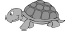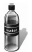# User Forum

Subject :IMO    Class : Class 6

Class : Class 7
6w

## Ans 2:

Class : Class 7
Breadth=w, Length is 5 times more, Therefore, length = 5w Therefore, perimeter=2(5w+w) =2*6w =12w Hence, b is the correct answer

Class : Class 6

Class : Class 4

## Ans 5:

Class : Class 7
P=l+b×2 L=5w B=w =5w+w×2 =6w×2 =12w cm

Subject :IMO    Class : Class 6

Class : Class 6
yeah....crrct

## Ans 2:

Class : Class 6
yeah

Class : Class 1

Subject :IMO    Class : Class 6

Class : Class 6

## Ans 2:

Class : Class 5
the answer can be 31 and 13

## Ans 3:

Class : Class 8
yes, and should be 32, which is not an option. Pl. correct this

Class : Class 5

## Ans 5:

Class : Class 6
Ya there is a problem...............answer is 32 you seee

Class : Class 7

## Ans 7:

Class : Class 6
There is problem in the Q as it mentions division sign represents both addition and substraction

## Ans 8:

Class : Class 7
yes there is a problem in the question

## Ans 9:

Class : Class 8
actually! it is 32...........plz correct it

Class : Class 7

## Ans 11:

Class : Class 8
the division sign represents both addition and subtraction and we can get to different answers

## Ans 12:

Class : Class 8
Yes there is a problem in the question

## Ans 13:

Class : Class 7
Then the calculation will turn to 20 +8/4x3x2, =20+2x3x2, =20+6x2, =20+12 =32, so there is a problem in the question

## Ans 14:

Class : Class 6
yes pls correct the problem...

## Ans 15:

Class : Class 7

Subject :IMO    Class : Class 10

## Ans 1:

Class : Class 10
C

Class : Class 1

In ΔAED
AE D + ∠EAD + 90° = 180° (Angle sum property)
⇒ ∠AED + ∠EAD = 90°
⇒ ∠EAD = 90° – ∠AED ...(i)
Also, ∠EAD = (50° + ∠AFB) ...(ii) (Exterior angle property)
From (i) and (ii), we have
50° + ∠AFB = 90° – ∠AED
⇒ ∠AFB + ∠AED = 90° – 50° = 40°
⇒ ∠AED + ∠CFD = 40°

Subject :IMO    Class : Class 5

Class : Class 5

## Ans 2:

Class : Class 5
D

Subject :IMO    Class : Class 6

Class : Class 1

In the 1st column, 2 X 1 + 1 = 3,

In the 2nd column, 12 X 9 + 9 = 117

Similarly, In the 3rd column, 7 X 14 + 7 = 105

Hence, the missing number is 7.

## Ans 2:

Class : Class 6

Subject :IMO    Class : Class 6

Class : Class 7
No idea

Class : Class 7
No idea

Class : Class 7
No idea

Class : Class 6

Class : Class 6
C is the ans

Class : Class 6

## Ans 7:

Class : Class 7
either a or d

Subject :IMO    Class : Class 4

## Ans 1:

Class : Class 3
Check the numbers then find the 4 in the ten thousand place

Class : Class 4
all sports

Class : Class 5
all sports

Class : Class 4
All sports

Class : Class 4

## Ans 6:

Class : Class 5
very easy bro! the answer is a (all sports)

Subject :IMO    Class : Class 5

Class : Class 7

Class : Class 7

## Ans 3:

Class : Class 5
D

Subject :IMO    Class : Class 2

## Ans 1:

Class : Class 4

Class : Class 1

Fourth from the left end is.

Third to the right ofis.

Class : Class 4